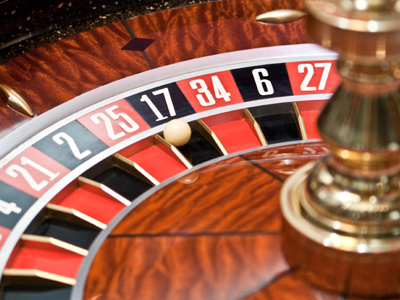This is a tricky one so think before you choose your answer!

# The 17 Times Table

If you want to do well in maths it's a good idea to learn the times tables off by heart. Most people learn the times tables up to 12 but, for KS3 Maths, we thought you'd like a bit more of a challenge. That's why we're going to test your times table knowledge all the way up to the 20 times table. In this quiz we look at the 17 times table.

34, 221 and 289 are answers found in the 17 times table. That's because they're all multiples of 17. Maths is of increasing importance in the modern world. As the mathematician and computer scientist, John Kemeny, said "The man ignorant of mathematics will be increasingly limited in his grasp of the main forces of civilisation."

If Kemeny is right then you'd better put your thinking cap on and improve your knowledge of the times tables! See how well you can do in this quiz all about the 17 times table.

1.
What is 18 x 17
308
302
298
306
2 x 17 = 34 so 20 x 17 = 340. Therefore, 340 - 34 = 18 x 17
2.
What is 20 x 17
344
340
339
348
2 x 17 = 34 so 20 x 17 = 340
3.
What is 15 x 17
252
255
248
265
10 x 17 = 170 and 5 x 17 = 85 so 170 + 85 = 15 x 17
4.
What is 2 x 17
32
33
34
38
34 has only 4 factors: 1, 2, 17 and 34
5.
What is 17 x 17
289
286
294
296
17 is the square root of 289
6.
What is 16 x 17
262
270
272
271
15 x 17 = 255 and 255 + 17 = 272
7.
What is 14 x 17
235
244
228
238
15 x 17 = 255 and 255 - 17 = 238
8.
What is 6 x 17
102
96
103
98
102 has 8 factors: 1, 2, 3, 6, 17, 34, 51 and 102
9.
What is 13 x 17
224
227
221
225
10 x 17 = 170 and 3 x 17 = 51 so 13 x 17 = 170 - 51
10.
What is 4 x 17
74
67
61
68
5 x 17 = 85 and 85 - 17 = 68
Author:  Frank Evans